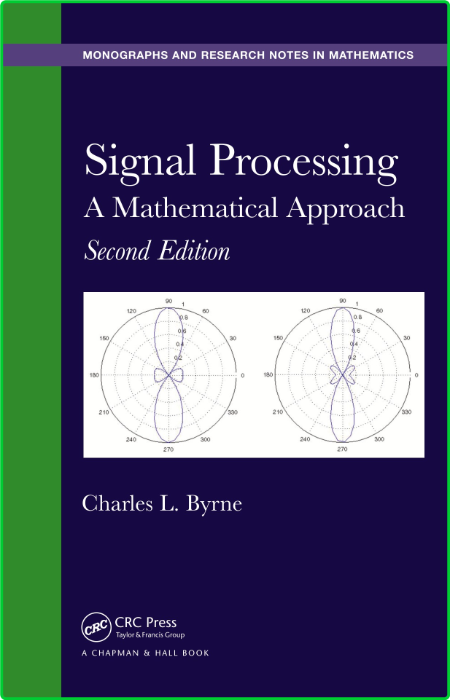# Signal Processing - A Mathematical Approach, Second Edition

Posted in: eBooks | By: Jocker | 19-07-2021, 12:26 | 0 Comments
19
July
2021Signal Processing - A Mathematical Approach, Second Edition (True PDF)
pdf | 4.59 MB | English | Isbn:‎ 978-0367658946 | Author: Charles L. Byrne | Year: 2020

Description:

Signal Processing: A Mathematical Approach is designed to show how many of the mathematical tools the reader knows can be used to understand and employ signal processing techniques in an applied environment. Assuming an advanced undergraduate- or graduate-level understanding of mathematics―including familiarity with Fourier series, matrices, probability, and statistics―this Second Edition:

• Contains new chapters on convolution and the vector DFT, plane-wave propagation, and the BLUE and Kalman filters
• Expands the material on Fourier analysis to three new chapters to provide additional background information
• Presents real-world examples of applications that demonstrate how mathematics is used in remote sensing

Featuring problems for use in the classroom or practice, Signal Processing: A Mathematical Approach, Second Edition covers topics such as Fourier series and transforms in one and several variables; applications to acoustic and electro-magnetic propagation models, transmission and emission tomography, and image reconstruction; sampling and the limited data problem; matrix methods, singular value decomposition, and data compression; optimization techniques in signal and image reconstruction from projections; autocorrelations and power spectra; high-resolution methods; detection and optimal filtering; and eigenvector-based methods for array processing and statistical filtering, time-frequency analysis, and wavelets.

• Category:Physics of Entropy, Telecommunications & Sensors, Internet & Telecommunications